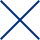Close

# diffraction aberration, diffraction limit

diffraction aberration, diffraction limit

The diffraction aberration causes a spread of an electron beam on the focal plane of the objective lens due to the wave nature of electrons. This term is also called "diffraction limit".

Electrons possess the wave nature and causes diffraction phenomena as light does. For the objective lens of the SEM, a circular objective aperture is used to decrease spherical and chromatic aberrations. Since the size of the aperture is finite, a diffraction phenomenon occurs. That is, the electron beam running parallel to the optical axis does not converge onto a point on the focal plane but forms an interference pattern, which is composed of a light disk around the optical axis (Airy disk) and plural circular rings (Airy pattern).
Fig. 1 shows the intensity distribution of the electron probe on the focal plane. The radius of the Airy disk (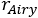) is expressed as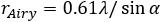. Here, α is the convergence semi-angle of the electron beam and usually 1 to 20 mrad. (If the distance between the objective lens and specimen (working distance, WD) is small, α becomes large, and vice vasa.) λ is the electron wave length. The wavelength is expressed as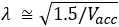(nm), where Vacc is the accelerating voltage of the electrons. The electron probe diameter dd on the focal plane is approximated to be the radius of Airy disk or dd =. Since α for SEM is usually 20 mrad or less, sin αα and then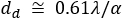.
Fig. 2 shows the accelerating voltage dependence of the electron probe diameter (dd) caused by diffraction. The electron probe diameter becomes large as the accelerating voltage becomes low or the electron wavelength λ becomes long. It is noted that the probe diameter rapidly increases below 5 kV. In the case of α = 5.0 mrad, dd is 1.1 nm for 20 kV, and dd is 4.7 nm for 1 kV.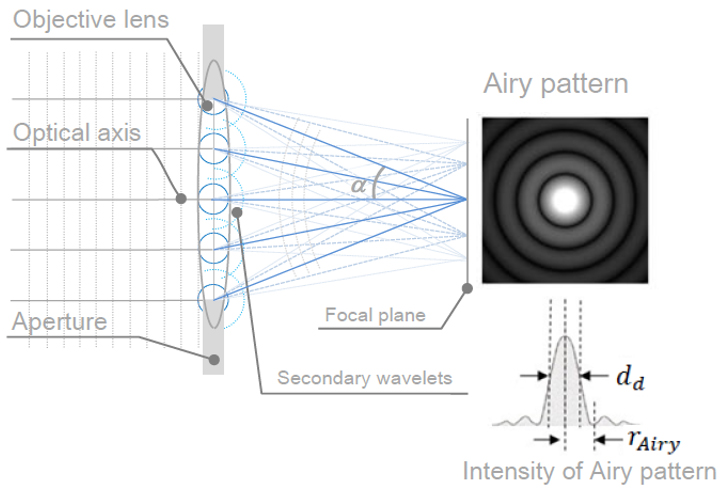Fig. 1 Schematic diagram of the spread of an electron beam on the focal plane due to diffraction.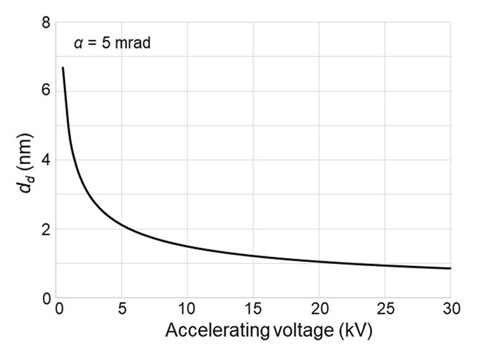Fig. 2 Accelerating voltage dependence of the electron probe diameter (dd)